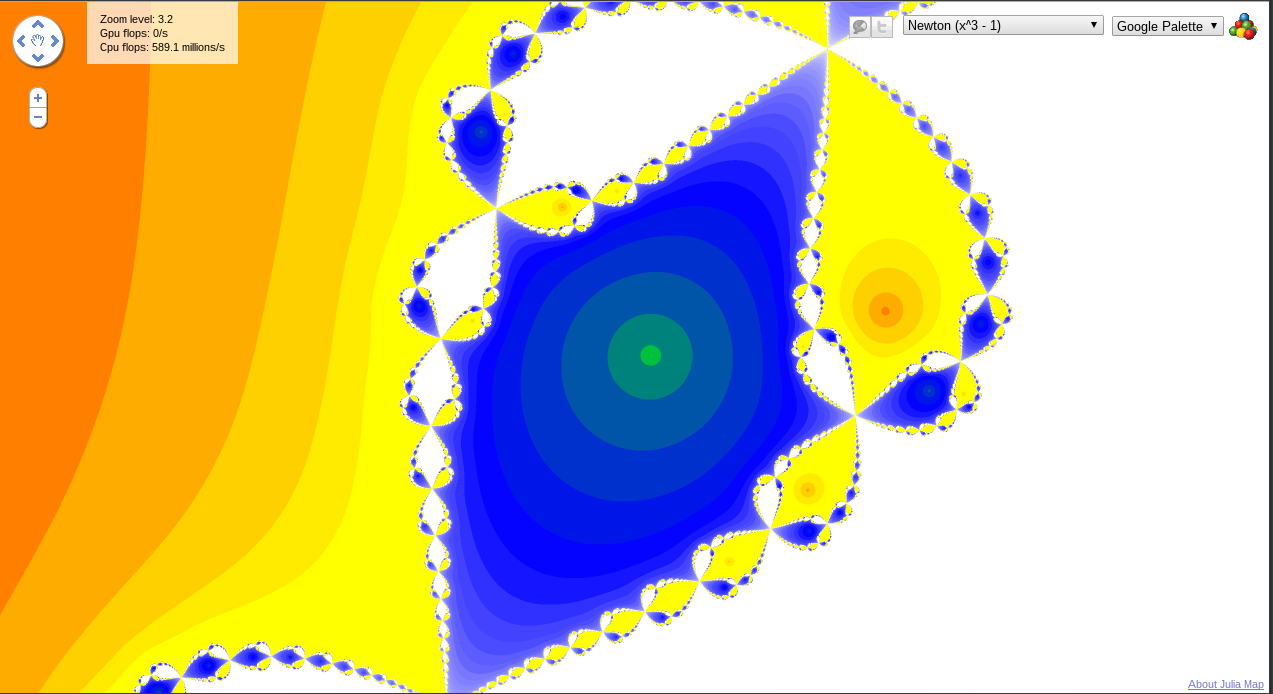In :
import numpy as np
import matplotlib.pyplot as plt
import svgwrite as svg
from IPython.display import Image
from IPython.display import SVG
import random

%pylab inline

Populating the interactive namespace from numpy and matplotlib

WARNING: pylab import has clobbered these variables: ['random']
%matplotlib prevents importing * from pylab and numpy


0. Go to the googlelabs.com Julia site that’s listed on the course webpage and zoom into the Newton’s method. Find some really interesting area and turn in a plot of it.

In :
Image(filename='fractal.png')

Out:1. Calculate (paper and pencil, not code) the capacity dimension for a middle-sixth-removed Cantor set.

Let's first draw what this Cantor set would look like.

In :
def cantorset(dwg, iterations, fraction, x0, y0, width):
if iterations == 0:
return
x, y = x0 + width, y0
dwg.add(dwg.line((x0, y0), (x, y), stroke=svg.rgb(0, 0, 0, '%'), stroke_width=2))
cantorset(dwg, iterations - 1, 1/6, x0, y0 + 5, (5 / 12) * width)
cantorset(dwg, iterations - 1, 1/6, x0 + ((5 / 12) * width) + ((1 / 6) * width),
y0 + 5, (5 / 12) * width)

dwg = svg.Drawing('cantor.svg', size=('10cm', '10cm'), profile='full')
dwg.viewbox(width=500, height=50)
lines = cantorset(dwg, 10, 1/6, 10, 10, 400)
dwg.save()
SVG(filename='cantor.svg')

Out:

Now lets look at how the number of line segments increases. As with the original cantor set, it remains $2^n$, however the length has now changed and is ${\left( \frac{5}{12} \right)}^n$.

Using the basic dimension formula:

$$n = \frac{1}{s^D}$$

Where $n$ is the number of elements and $s$ is the scaling factor we plug in our values and solve for $D$.

\begin{aligned} 2 &=& \frac{1}{{\left( \frac{5}{12} \right)}^D}\\ 2 &=& {\left( \frac{12}{5} \right)}^D\\ \log_{12 / 5} 2 &=& D\\ \frac{\ln 2}{\ln \frac{12}{5}} &=& D\\ \end{aligned}

Solving for a numerical approximation to $D$ we obtain

In :
np.log(2) / np.log(12/5)

Out:
0.79174406918855766

2. a) Transform the following third-order ODE into three first-order ODEs.

$$2 x^{\prime\prime\prime}(t) - 3 \tan \left( \frac{1}{2} \cdot x^{\prime\prime}(t) \right) + 16 \log\left( x^\prime(t) \right) - x(t) = 0$$

We start by rewriting this to put the third order derivative on the left hand side.

$$x^{\prime\prime\prime}(t) = \frac{3 \tan \left( \frac{1}{2} \cdot x^{\prime\prime}(t) \right) - 16 \log\left( x^\prime(t) \right) + x(t)}{2}$$

Now we set three helper variables, which we will define as the following.

$$y_1 = x, y_2 = x^\prime, y_3 = x^{\prime\prime}$$

Using these we can rewrite the equation into several and take the derivative of each equation.

\begin{aligned} y_1^\prime &= x^\prime = y_2\\ y_2^\prime &= x^{\prime\prime} = y_3\\ y_3^\prime &= x^{\prime\prime\prime} = \frac{3 \tan \left( \frac{1}{2} \cdot x^{\prime\prime}(t) \right) - 16 \log\left( x^\prime(t) \right) + x(t)}{2} = \frac{3 \tan \left( \frac{1}{2} \cdot y_3(t) \right) - 16 \log\left( y_2(t) \right) + y_1}{2} \end{aligned}

Yielding our final three equations.

$$\begin{cases} y_1^\prime &= y_2\\ y_2^\prime &= y_3\\ y_3^\prime &= \frac{3 \tan \left( \frac{1}{2} \cdot y_3(t) \right) - 16 \log\left( y_2(t) \right) + y_1}{2} \end{cases}$$

b) Transform the following set of first-order ODEs into a single higher-order ODE.

\begin{aligned} \dot{x} &=& y\\ \dot{y} &=& z\\ \dot{z} &=& yz + \log y \end{aligned}

Simply substituting in the "helper variables" we can come back to the original equation.

$$x^\prime x^{\prime\prime} + \log x^\prime = x^{\prime\prime\prime}$$

c) Are the systems in parts (a) and (b) of this problem linear or nonlinear? Why?

These systems are nonlinear because they have nonlinear functions associated with them, namely $\tan$ and $\log$.

3. a) Write a program that begins with a vertical line segment, then iterates the following operation: draw two line segments at right angles from the top of the previous segment, each $0.6$ times the length of that segment. Iterate until you can no longer discern differences between successive segments. Turn in a plot.

In :
def fractalate(dwg, iterations, x0, y0, length, reduction, init_angle, branch_angle):
if iterations == 0:
return
x = x0 + length * np.cos(init_angle)
y = y0 + length * np.sin(init_angle)
dwg.add(dwg.line((x0, y0), (x, y), stroke=svg.rgb(0, 0, 0, '%'), stroke_width=1))
fractalate(dwg, iterations - 1, x, y, length * reduction, reduction,
init_angle + branch_angle, branch_angle)
fractalate(dwg, iterations - 1, x, y, length * reduction, reduction,
init_angle - branch_angle, branch_angle)

dwg = svg.Drawing('fractaltree.svg', size=('20cm', '20cm'), profile='full')
dwg.viewbox(width=1000, height=1000)
lines = fractalate(dwg, 12, 500, 1000, 500, 0.6, -np.pi / 2, np.pi / 2)
dwg.save()
SVG(filename='fractaltree.svg')

Out:

b) What happens to the tree if each segment is exactly half as long as the previous one? What if it is larger than $0.707$?

Looking at the plots below we see the behavior differs in each case. In the first case, the "decay" rate, the rate at which the iterations are no longer distinguishable increases substantially and after only a few iterations we can no longer discern the difference between successive iterations.

For the other case, for when it is larger than $0.7$, the decay rate isn't large enough and the "branches" become intertwined to the point of no longer being able to discern one branch from the other.

In other words, in order to have a "nice" looking fractal binary tree, there is a very small range of values in which the scaling factor must lie.

In :
dwg = svg.Drawing('fractaltree0.5.svg', size=('20cm', '20cm'), profile='full')
dwg.viewbox(width=1000, height=1000)
lines = fractalate(dwg, 10, 500, 1000, 500, 0.5, -np.pi / 2, np.pi / 2)
dwg.save()
SVG(filename='fractaltree0.5.svg')

Out:
In :
dwg = svg.Drawing('fractaltree0.3.svg', size=('20cm', '20cm'), profile='full')
dwg.viewbox(width=1000, height=1000)
lines = fractalate(dwg, 10, 500, 1000, 500, 0.3, -np.pi / 2, np.pi / 2)
dwg.save()
SVG(filename='fractaltree0.3.svg')

Out: